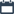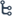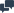## Thursday, February 27, 2020

•February 27, 2020
••, , ,
•No comments

## A book seller has 175 English books, 245 Science books and 385 Mathematics books. He wants to sell the books in a box, subject-wise in equal numbers. What will be the greatest number of the boxes required? Also find the number of books for each subject in a box.

A book seller has 175 English books, 245 Science books and 385 Mathematics books. He wants to sell the books in a box, subject-wise in equal numbers. What will be the greatest number of the boxes required? Also find the number of books for each subject in a box.
Answer : the total number of books in each box is 5+7+11=23.

## Categories